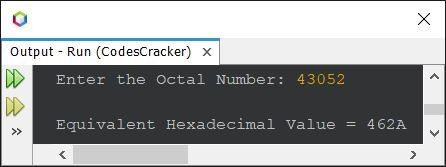# Java Program to Convert Octal to Hexadecimal

## Octal to Hexadecimal using Function in Java

The question is, write a Java program to convert octal to hexadecimal using inbuilt function. The program given below is its answer. I've used some inbuilt functions of Java to do the job in shortcut way.

```import java.util.Scanner;

public class CodesCracker
{
public static void main(String[] args)
{
String octnum, hexnum;
int decnum;
Scanner scan = new Scanner(System.in);

System.out.print("Enter the Octal Number: ");
octnum = scan.nextLine();

decnum = Integer.parseInt(octnum, 8);
hexnum = Integer.toHexString(decnum).toUpperCase();

System.out.println("\nEquivalent Hexadecimal Value = " +hexnum);
}
}```

The snapshot given below shows the sample run of above program with user input 43052 as octal number to convert and print its equivalent hexadecimal value:In above program, using the following statement:

`decnum = Integer.parseInt(octnum, 8);`

the octal value stored in octnum gets converted into its equivalent decimal value, then initialized to decnum. And using the statement:

`hexnum = Integer.toHexString(decnum).toUpperCase();`

the decimal value stored in decnum gets converted into its equivalent hexadecimal and capitalized the hex characters (if available) using toUpperCase(), and initialized to hexnum. And finally using the last statement, the equivalent hexadecimal value of given octal number, gets printed on the output screen. That's it.

You can refer to Octal to Decimal in Java and Decimal to Hexadecimal in Java to combine and implement the manual or user-based code to convert octal to hexadecimal. Try with yourself. If any error or something goes wrong, then consider the program given below.

## Octal to Hexadecimal without using Function in Java

This program is created using user-based code. In this program, I've converted the entered octal value to decimal, and then decimal value gets converted into hexadecimal.

```import java.util.Scanner;

public class CodesCracker
{
public static void main(String[] args)
{
int octal, decimal=0, i=0, rem;
Scanner s = new Scanner(System.in);

System.out.print("Enter the Octal Number: ");
octal = s.nextInt();

// converting octal to decimal
while(octal!=0)
{
rem = octal%10;
decimal = (int) (decimal + (rem*Math.pow(8, i)));
i++;
octal = octal/10;
}

while(decimal!=0)
{
rem = decimal%16;
if(rem<10)
rem = rem+48;
else
rem = rem+55;
i++;
decimal = decimal/16;
}

// printing the equivalent hexadecimal value
for(i=(i-1); i>=0; i--)
}
}```

Since this program does the same job as of previous program, therefore you'll get the same output, of course.

#### Same Program in Other Languages

Java Online Test

« Previous Program Next Program »